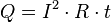# Joule's laws facts for kids

Kids Encyclopedia Facts

Joule's laws are two: first about heat produced by an electric current, and second about how the energy of a gas relates to pressure, volume.

Joule's first law shows the relation between heat generated by an electric current flowing through a conductor. It is named after James Prescott Joule and shown as:$Q = I^2 \cdot R \cdot t$

Where Q is the amount of heat, I is the electric current flowing through a conductor, R is the amount of electric resistance present in the conductor, and t is the amount of time that this happens for.

Joule's second law says that the internal energy of an ideal gas does not change if volume and pressure change, but does change if temperature changes.

Joule's law is important.Joule's laws Facts for Kids. Kiddle Encyclopedia.